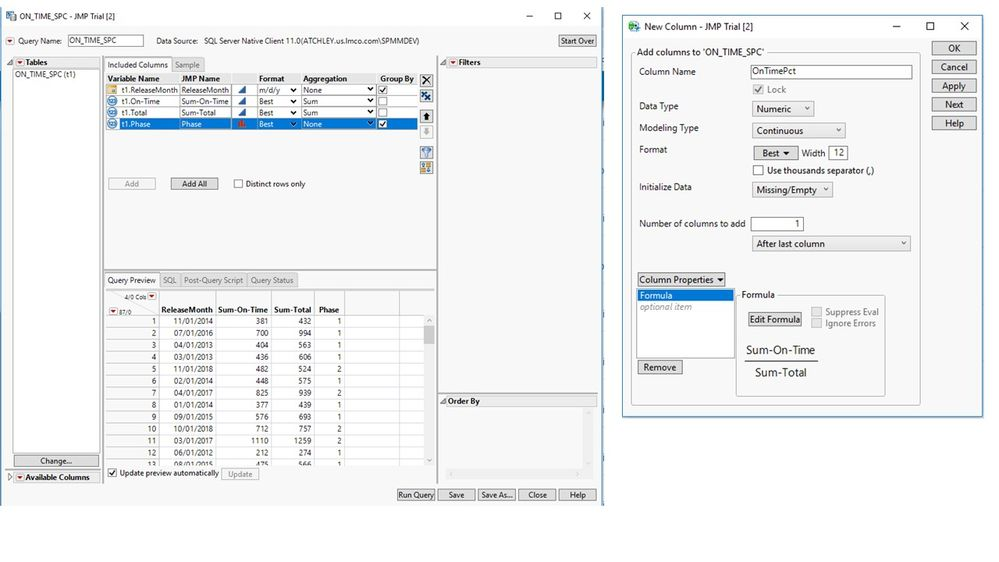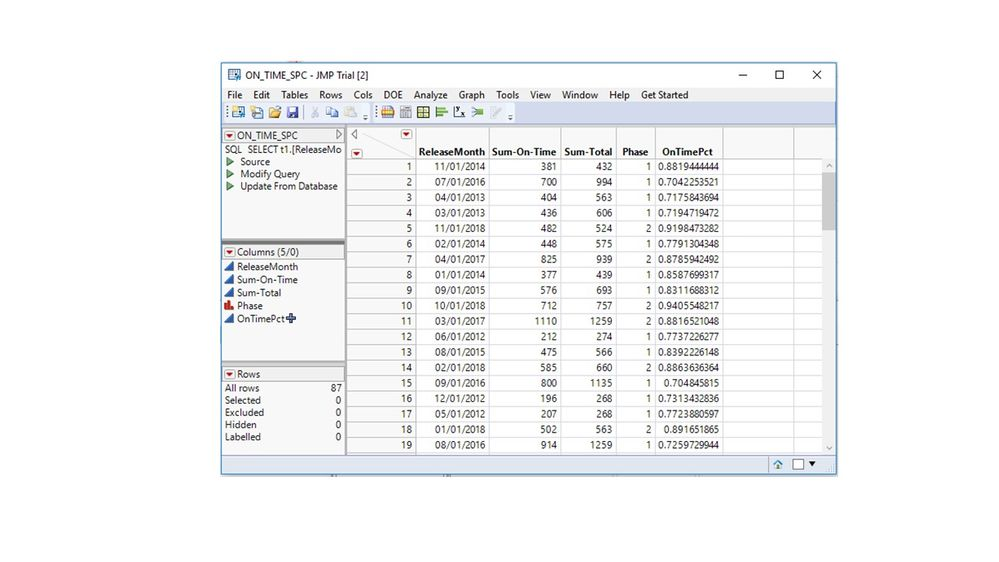Our World Statistics Day conversations have been a great reminder of how much statistics can inform our lives. Do you have an example of how statistics has made a difference in your life? Share your story with the Community!
Choose Language Hide Translation Bar
Highlighted

## JMP Table Script Not Including Column Expression

I'm trying to automate my JMP chart creation. I've been using  the following as a guide: Automated Report Creation From Data Import to Publication 2019

I've gotten to the point where I create the table from a SQL Query. I had to add a new column to the table which is an expression. The issue is that I can't get the script to show this calculated column. When I right click "Modify Query" it only shows the base query and not the calculated column. How do I get the script that includes the calculated column?

1 ACCEPTED SOLUTION

Accepted Solutions
Highlighted

## Re: JMP Table Script Not Including Column Expression

The table scripts are created with the result of the query. They do not capture any changes to the data table afterward such as adding the data column with the ratio calculation.

I added the code to create this column for you and appended it to the end of the Modify Query script. It is for proof of principle.

``````Names Default To Here( 1 );

dt = New SQL Query(
Connection( "CONNECTION HERE" ),
QueryName( "ON_TIME_SPC" ),
Select(
Column( "ReleaseMonth", "t1", Numeric Format( "m/d/y", "-1", "NO", "" ) ),
Column(
"On-Time",
"t1",
Alias( "Sum-On-Time" ),
SavedJMPName( "On-Time" ),
Aggregation( "Sum" ),
JMP Name( "Sum-On-Time", 0 )
),
Column(
"Total",
"t1",
Alias( "Sum-Total" ),
SavedJMPName( "Total" ),
Aggregation( "Sum" ),
JMP Name( "Sum-Total", 0 )
),
Column( "Phase", "t1", Analysis Type( "Nominal" ) )
),
From( Table( "ON_TIME_SPC", Schema( "dbo" ), Alias( "t1" ) ) ),
Group By(
Column( "ReleaseMonth", "t1", Numeric Format( "m/d/y", "-1", "NO", "" ) ),
Column( "Phase", "t1" )
)
);

dt << New Column( "OnTimePct",
Formula( :Name( "Sum-On-Time" ) / :Name( "Sum-Total" )
);``````

I save a reference to the new data table in the variable dt so I can send messages. I send the << New Column() message to create the column with the ratio.

Learn it once, use it forever!
4 REPLIES 4
Highlighted

## Re: JMP Table Script Not Including Column Expression

"I had to add a new column to the table which is an expression." What is the expression? Code to add a new column?

"I can't get the script to show this calculated column." Show in the data grid or the columns panel? Is the data column hidden to begin with?

"When I right click "Modify Query" it only shows the base query and not the calculated column." Isn't the Modify Query table script created automatically with the query results? Did you attempt to edit it manually or with code?

"How do I get the script that includes the calculated column?" You generally send the << Get Script message to any object, such as a data column or a data table.

Can you share your current version of the script?

Learn it once, use it forever!
Highlighted

## Re: JMP Table Script Not Including Column Expression

1. The calculated field is Sum(OnTime)/Sum(Total).

2. These are the steps I take.

• Open table via query builder
• Add columns I need and then select "Run Query"
• I couldn't add my calculated field at Query Builder because it would automatically set the "Group By" column and I couldn't deselect it
• It also wouldn't allow me to manually write a query
• On the table that comes up I can add a new column based on a calculation that shows in the table, HOWEVER
• Modify Query ONLY shows the original script that created the table and not the script for the calculated column

3. I would attempt to modify the script to add the calculated column, but have no idea what the syntax is. Here are some screen shots:New SQL Query( Connection( "CONNECTION HERE" ), QueryName( "ON_TIME_SPC" ), Select( Column( "ReleaseMonth", "t1", Numeric Format( "m/d/y", "-1", "NO", "" ) ), Column( "On-Time", "t1", Alias( "Sum-On-Time" ), SavedJMPName( "On-Time" ), Aggregation( "Sum" ), JMP Name( "Sum-On-Time", 0 ) ), Column( "Total", "t1", Alias( "Sum-Total" ), SavedJMPName( "Total" ), Aggregation( "Sum" ), JMP Name( "Sum-Total", 0 ) ), Column( "Phase", "t1", Analysis Type( "Nominal" ) ) ), From( Table( "ON_TIME_SPC", Schema( "dbo" ), Alias( "t1" ) ) ), Group By( Column( "ReleaseMonth", "t1", Numeric Format( "m/d/y", "-1", "NO", "" ) ), Column( "Phase", "t1" ) ) )

Highlighted

## Re: JMP Table Script Not Including Column Expression

The table scripts are created with the result of the query. They do not capture any changes to the data table afterward such as adding the data column with the ratio calculation.

I added the code to create this column for you and appended it to the end of the Modify Query script. It is for proof of principle.

``````Names Default To Here( 1 );

dt = New SQL Query(
Connection( "CONNECTION HERE" ),
QueryName( "ON_TIME_SPC" ),
Select(
Column( "ReleaseMonth", "t1", Numeric Format( "m/d/y", "-1", "NO", "" ) ),
Column(
"On-Time",
"t1",
Alias( "Sum-On-Time" ),
SavedJMPName( "On-Time" ),
Aggregation( "Sum" ),
JMP Name( "Sum-On-Time", 0 )
),
Column(
"Total",
"t1",
Alias( "Sum-Total" ),
SavedJMPName( "Total" ),
Aggregation( "Sum" ),
JMP Name( "Sum-Total", 0 )
),
Column( "Phase", "t1", Analysis Type( "Nominal" ) )
),
From( Table( "ON_TIME_SPC", Schema( "dbo" ), Alias( "t1" ) ) ),
Group By(
Column( "ReleaseMonth", "t1", Numeric Format( "m/d/y", "-1", "NO", "" ) ),
Column( "Phase", "t1" )
)
);

dt << New Column( "OnTimePct",
Formula( :Name( "Sum-On-Time" ) / :Name( "Sum-Total" )
);``````

I save a reference to the new data table in the variable dt so I can send messages. I send the << New Column() message to create the column with the ratio.

Learn it once, use it forever!
Highlighted